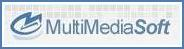﻿ Methods > Init and info > OutputVolumeGet methodCopyright © 2011-2018 MultiMedia Soft OutputVolumeGet methodRemarks

Retrieves the current volume. The volume can be changed through the OutputVolumeSet method.

Syntax

 [Visual Basic] Public OutputVolumeGet ( nScaleType ) as float

 [C#] public float OutputVolumeGet ( nScaleType );

 [C++] public: float OutputVolumeGet ( nScaleType );

Parameter

Description

nScaleType

The volume scaling type.

Supported values are the following:

 Mnemonic constant Value Meaning SCALE_LINEAR 0 The returned volume is based upon a linear scale and can be in the range from 0.0 (silence) to 100.0 (max volume) SCALE_LOG 1 The returned volume, expressed in dB, is based upon a logarithmic scale and can be in the range from -100  (silence) to 0 (max volume) The actual applied formula for converting a linear volume into a logarithmic volume is the following: VolumeInDB = 20 * log10 (VolumeLinear/100) The C syntax for converting a logarithmic volume into a linear volume is the following: VolumeLinear = 100 * pow (10, VolumeInDB/20);

Return value

 Value Meaning Negative value An error occurred (see the LastError property for further error details) >= 0 The current volume